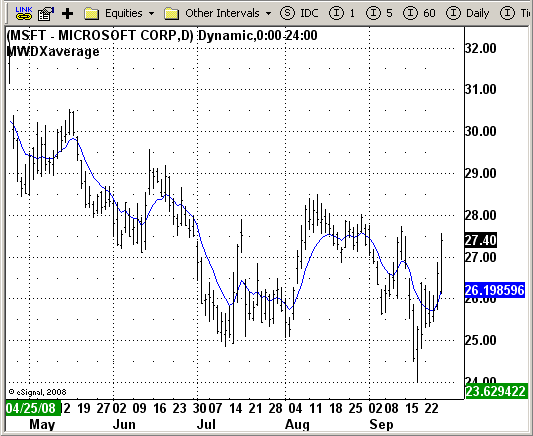# MWD XAverage

ICE Data Services -

MWDXAverage.efs
EFSLibrary - Discussion Board

File Name: MWDXAverage.efs

Description:
MWD XAverage indicator

Formula Parameters:

• Moving average source: close
• Smoothing factor: 0.2

Notes:

MWD Xaverage an exponential moving average indicator which uses a [0,1] smoothing factor. The moving average length is calculated from the smoothing factor according to formula:

`LENGTH = 2/FAC - 1;`

MWDXAverage.efsEFS Code:

```/*********************************

Provided By:

eSignal (Copyright c eSignal), a division of Interactive Data
Formula Script (EFS) is for educational purposes only and may be
modified and saved under a new file name.  eSignal is not responsible
for the functionality once modified.  eSignal reserves the right
to modify and overwrite this EFS file with each new release.

Description:

MWD XAverage indicator.

Version:            1.0  09/26/2008

Notes:

MWD Xaverage an exponential moving average indicator which uses
a [0,1] smoothing factor. The moving average length is calculated
from the smoothing factor according to formula:
LENGTH = 2/FAC - 1;

Formula Parameters:                     Default:

Moving average source               close
Smoothing factor                    0.2

**********************************/

var fpArray = new Array();

function preMain() {
setPriceStudy(true);
setStudyTitle("MWDXaverage");
setCursorLabelName("MWXAvg", 0);

var x=0;
fpArray[x] = new FunctionParameter("MASource", FunctionParameter.STRING);
with(fpArray[x++]) {
setName("Source of moving average");
setDefault("close");
}

fpArray[x] = new FunctionParameter("nFAC", FunctionParameter.NUMBER);
with(fpArray[x++]) {
setName("Factor");
setLowerLimit(0.01);
setDefault(0.2);
}
}

var xPrice = null;
var xMWDX = null;
var bInit = false;

function main(MASource, nFAC) {

var nBarState = getBarState();
var nBarCount = getCurrentBarCount();

if(nBarState == BARSTATE_ALLBARS) {
if (MASource == null) MASource = "close";
if (nFAC == null) nFAC = 0.2;
}

if(bInit == false) {
xPrice = eval(MASource)();
xMWDX = ema(Math.round(2/nFAC-1), xPrice);
bInit = true;
}

var nValue1 = xMWDX.getValue(0);
return nValue1;
}```